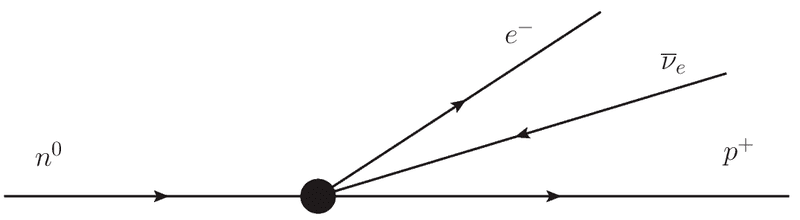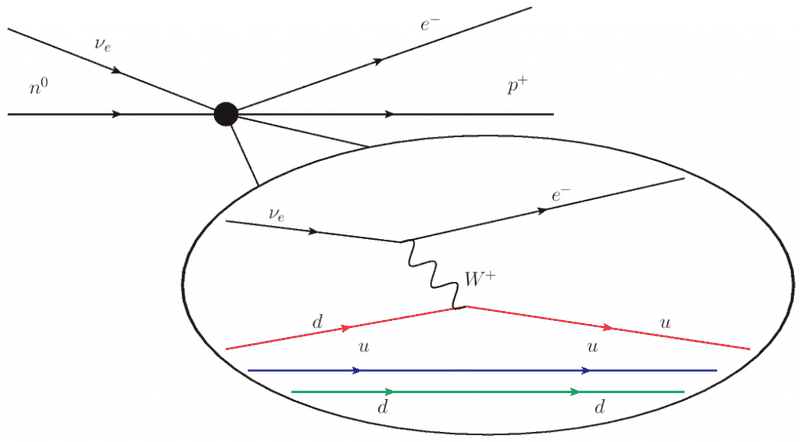# Why exactly three lines at a Feynman vertex?

Staff Emeritus
Gold Member
The Feynman diagram you'd naively draw for a beta decay such as $p\rightarrow n + e^++\bar{\nu}$ would have four lines joined at a vertex, but in reality there's a virtual W or Z involved, which makes it into two 3-vertices.

Is there any fundamental reason why all the vertices in a field theory *must* be 3-line vertices, and not 4 or more? If we'd known about the general structure of field theory before the discovery of the neutrino, could we have inferred that there must be some intermediate particle such as the W or Z?

[EDIT] Googling on "charged scalar field," I get, e.g., a statement that in such a theory, "the number of arrows flowing into a vertex equals the number flowing out" (Sterman, p. 110). So I guess maybe there is no reason we can't have 4 or more lines at a vertex, and maybe my question should really be whether such things exist in the standard model, or if not, whether there is any known reason why the universe has to be this way.

Last edited:

Ah, but it's not always true. The Higgs interaction terms

L = |(∂μ - g ½τ·Wμ - g' ½YBμ)φ|2 - V(φ)

are quartic, and therefore lead to vertices in the Feynman diagrams having four legs.

Staff Emeritus
Gold Member
Ah, but it's not always true. The Higgs interaction terms

L = |(∂μ - g ½τ·Wμ - g' ½YBμ)φ|2 - V(φ)

are quartic, and therefore lead to vertices in the Feynman diagrams having four legs.

Aha, thanks! I'm not sophisticated about reading off properties of Lagrangians. What are the legs in this diagram? Four Higgses?

kurros
There are a whole heap of diagrams that come out of that Lagrangian. There is stuff hidden in V($\varphi$) which gets you the quartic Higgs self-interaction (hhhh) you mention, but also mixed in there are WWhh and ZZhh. You can vaguely see it if you imagine expanding the square, and there will be terms containing WW$\varphi$$\varphi$ and BB$\varphi$$\varphi$, which once you do the electroweak symmetry breaking basically lead to the diagrams I mention. I think the various 4 gauge boson coupling must come out of there also, stuff like WWWW, WWZZ, WWZγ and WWγγ, err even ZZγγ is in there I think...

There can, however, be no 4 legs vertices containing fermions, since you can't write down terms that do that without violating Lorentz invariance. Hmm actually that only takes care of diagrams with 2 fermions and 2 vectors, or 2 fermions + 2 scalars . Why not 2 fermions, 1 vector, and 1 scalar? Uh, can't think of a reason why not just now... maybe you can do it? They aren't in the Standard Model though...

As for diagrams with even more legs at one vertex, well my formal theory is not so good but if I remember correctly they are not renormalisable, for some reason I don't remember. So you can have them in effective theories, but they cause your theory to explode at high energy scale. Actually I think this is what disallows the 4-fermion interactions also, which do not violate Lorentz invariance either. The fermi theory of weak interactions has 4 fermion vertices after all.

Last edited:
Kraflyn
Hi.

Yes, the reason there are 3 legs is renormalizability. In some cases 4 legs may be allowed. Please allow me to explain. My story here is only for illustration; I won't bother to put in even $i\epsilon$ prescription, and I will omit free amplitudes since they don't affect the divergence or the convergence of the result.

Let's begin by calculating scattering amplitude for some well known process. This will demonstrate why we actually can calculate amplitude $\mathcal{M}$. Consider Feynman diagram in this image:

http://www-zeus.physik.uni-bonn.de/~brock/feynman/vtp_ws0506/chapter04/mupair.jpg

There are 2 vertices, so we put factor
$$(-ig)^2$$
as our starting integrand in impulse space.
There is 1 internal line, so here goes factor $i/q^2$. So now we have
$$(-ig)^2\frac{i}{q^2}$$
as our integrand.
We next notice conservation of 4-impulse in left vertex. So here goes factor $(2 \pi)^4 \delta^{(4)} (p_1 -p_2 -q)$. We have now arrived at
$$(2 \pi)^4 (-ig)^2\frac{i}{q^2} \delta^{(4)} (p_1 -p_2 -q)$$
as our integrand so far.
We next notice conservation of 4-impulse in right vertex. So here goes factor $(2 \pi)^4 \delta^{(4)} (p_4 +q-p_3 )$. We are now at
$$(2 \pi)^8 (-ig)^2\frac{i}{q^2} \delta^{(4)} (p_1 -p_2 -q) \delta^{(4)} (p_4 +q-p_3 )$$
We next integrate over internal line with measure $d^4 q /(2 \pi)^4$. Integral is
$$-i g^2 (2 \pi)^4 \int \frac{i}{q^2} \delta^{(4)} (p_1 -p_2 -q) \delta^{(4)} (p_4 +q-p_3 ) d^4 q$$
However, there is no actual integration required, because we have 2 delta functions inside our integral. After integrating over muon delta $(2 \pi)^4 \delta^{(4)} (p_4 +q-p_3 )$ and discarding the remaining delta, amplitude for muon is simply
$$\mathcal{M} = \frac{g^2}{(p_3 - p_4)^2}$$

We notice no integration was actually done, except the trivial one over delta function. If we had to integrate without delta functions, we'd be in trouble.

How much in trouble? Consider following image - Feynman diagram for electron self-energy, top left diagram:

http://physics.aps.org/assets/504e151caf028bb0 [Broken]

OK, first we find delta for left vertex. It is $(2 \pi)^4 \delta^{(4)} (p-p+l-l)= (2 \pi)^4 \delta^{(4)} (0) =\lim_{\Delta \to 0+}\frac{(2 \pi)^4}{\Delta^4}$. Ops... It diverges... What about right vertex? Same. So, we should integrate over internal line? With this? We have a divergent integral now even if we discarded both deltas!

So what about 2 vertices and 2 internal lines? Well, the integral we are left with is similar to our convergent integral we started with:
$$g^2 (2 \pi)^4 \int \!\!\! \int \frac{1}{q_1^2} \frac{1}{q_2^2} \delta^{(4)} (p_1 -p_2 -q_1 -q_2) \delta^{(4)} (p_4 +q_1 +q_2 -p_3 ) d^4 q_1 d^4 q_2$$
I just inserted another extra mediator, so now mediators have impulses $q_1$ and $q_2$.

OK, this is easy, we say. We first use first delta to the left, having $q_1 + q_2 = p_1 - p_2$. And now we are left with integral only over, say, $q_2$. The other delta left has argument $p_4 - p_3 +p_1 - p_2$ and now we can... Wait... This argument has no $q_2$ in it! And argument $p_4 - p_3 +p_1 - p_2$ is our conservation law: it is identically zero. So delta of it diverges the same way it did for electron self-energy. Same story again.

Oh yeah... It diverges alright.

So what happened? Well, some internal lines remained unchecked by delta. There is an elaborate theory about regularization and renormalization, though. The conclusion on renormalization is very simple. Feynman diagram with $f_E$ external fermionic legs and with $b_E$ external bosonic spin-1 legs will have convergent amplitude only if
$$b_E + \frac{3}{2}f_E >4$$
However, this is only true with 1 internal line per vertex pair. So what about 2 internal lines per 1 vertex pair? 1 vertex delta function cannot handle 2 integrations over 2 internal lines. It can only handle 1 integration. So 1 divergent integral will remain. Can we regularize it and renormalize it? Not with our contemporary knowledge, no.

And what about Fermi beta decay:It has four fermionic external legs. Hence $\frac{3}{2} 4 =6 > 4$ and it is a renormalizable process. There's a whole lot of shaking going on with internal lines in shaded area of actual interaction, of course, just as You mentioned.Finally, I'd like to add that I'm not quite sure I was answering the right question here :shy: So please have mercy on meCheers.

Last edited by a moderator:
Staff Emeritus